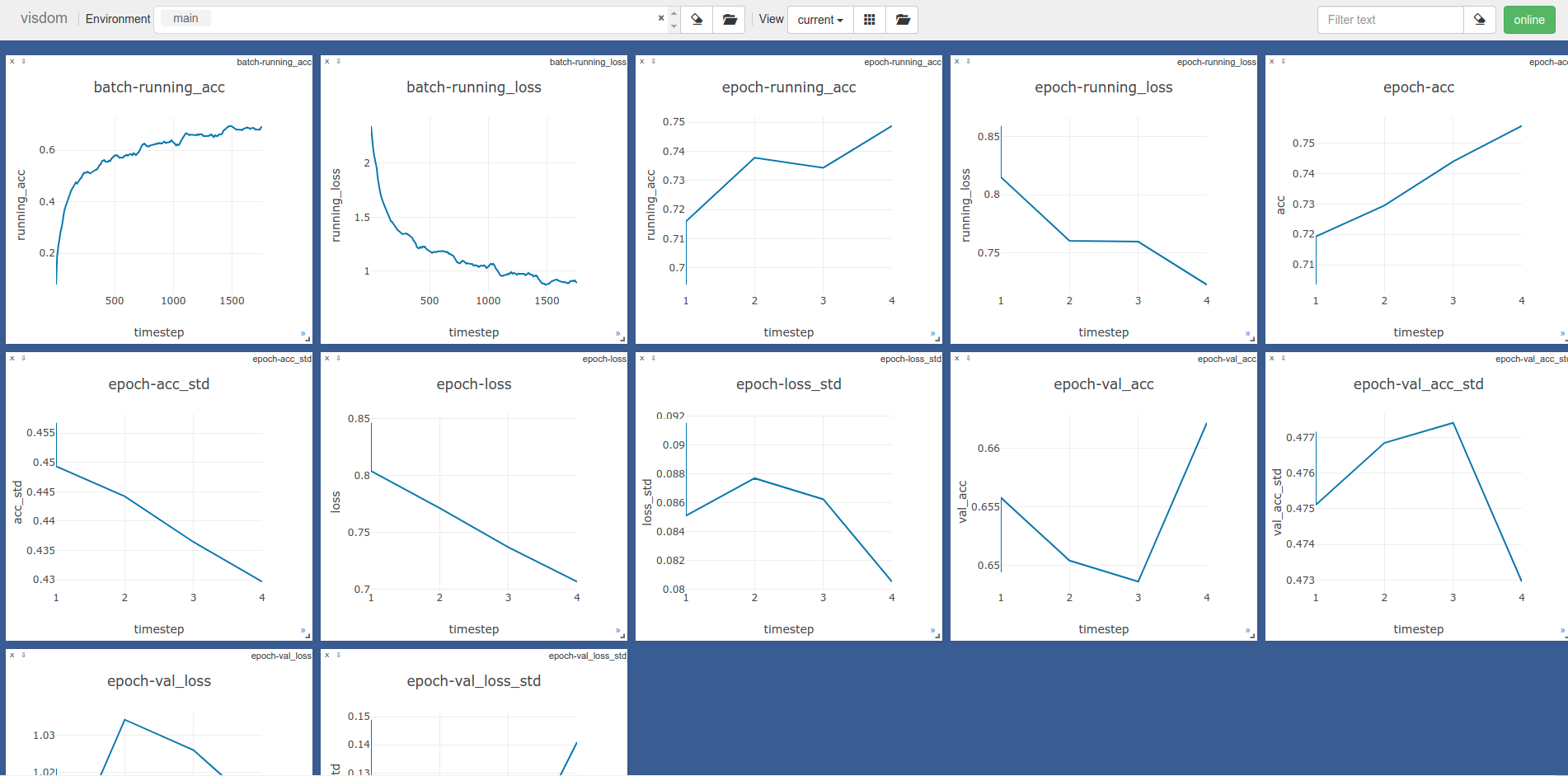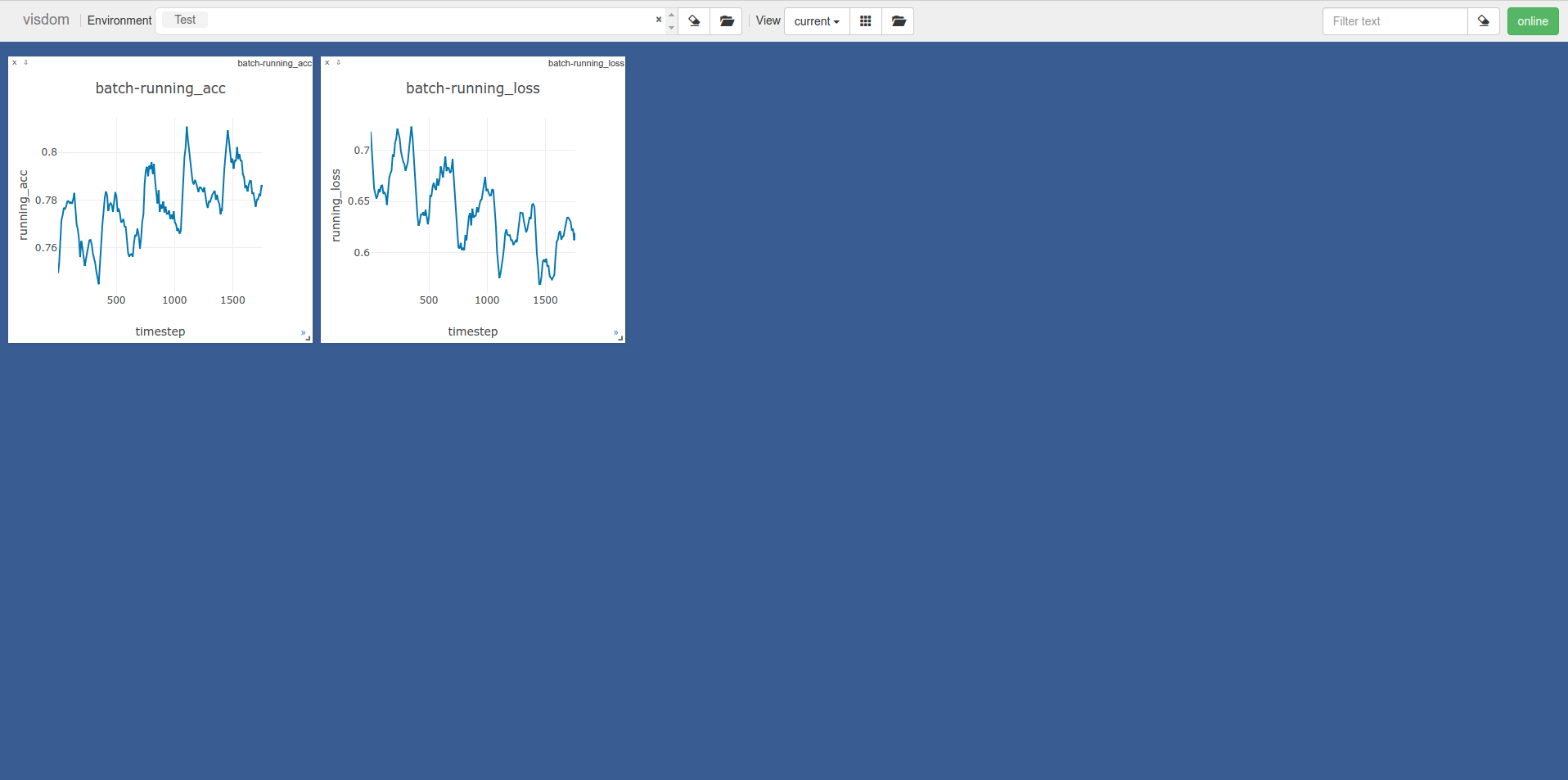# Logging to Visdom¶

In this note we will cover the use of the `TensorBoard callback` to log to visdom. See the tensorboard note for more on the callback in general.

## Model Setup¶

We’ll use the same setup as the tensorboard note.

```BATCH_SIZE = 128

normalize = transforms.Normalize(mean=[0.485, 0.456, 0.406],
std=[0.229, 0.224, 0.225])

transform=transforms.Compose([transforms.ToTensor(), normalize]))
splitter = DatasetValidationSplitter(len(dataset), 0.1)
trainset = splitter.get_train_dataset(dataset)
valset = splitter.get_val_dataset(dataset)

traingen = torch.utils.data.DataLoader(trainset, pin_memory=True, batch_size=BATCH_SIZE, shuffle=True, num_workers=10)
valgen = torch.utils.data.DataLoader(valset, pin_memory=True, batch_size=BATCH_SIZE, shuffle=True, num_workers=10)

transform=transforms.Compose([transforms.ToTensor(), normalize]))
testgen = torch.utils.data.DataLoader(testset, pin_memory=True, batch_size=BATCH_SIZE, shuffle=False, num_workers=10)
```
```class SimpleModel(nn.Module):
def __init__(self):
super(SimpleModel, self).__init__()
self.convs = nn.Sequential(
nn.Conv2d(3, 16, stride=2, kernel_size=3),
nn.BatchNorm2d(16),
nn.ReLU(),
nn.Conv2d(16, 32, stride=2, kernel_size=3),
nn.BatchNorm2d(32),
nn.ReLU(),
nn.Conv2d(32, 64, stride=2, kernel_size=3),
nn.BatchNorm2d(64),
nn.ReLU()
)

self.classifier = nn.Linear(576, 10)

def forward(self, x):
x = self.convs(x)
x = x.view(-1, 576)
return self.classifier(x)

model = SimpleModel()

loss = nn.CrossEntropyLoss()
```

## Logging Epoch and Batch Metrics¶

Visdom does not support logging model graphs so we shall start with logging epoch and batch metrics. The only change we need to make to the tensorboard example is setting `visdom=True` in the `TensorBoard callback` constructor.

```torchbearer_trial = Trial(model, optimizer, loss, metrics=['acc', 'loss'], callbacks=[TensorBoard(visdom=True, write_graph=True, write_batch_metrics=True, batch_step_size=10, write_epoch_metrics=True)]).to('cuda')
torchbearer_trial.with_generators(train_generator=traingen, val_generator=valgen)
torchbearer_trial.run(epochs=5)
```

If your visdom server is running then you should see something similar to the figure below:## Visdom Client Parameters¶

The visdom client defaults to logging to localhost:8097 in the main environment however this is rather restrictive. We would like to be able to log to any server on any port and in any environment. To do this we need to edit the `VisdomParams` class.

```class VisdomParams:
""" ... """
SERVER = 'http://localhost'
ENDPOINT = 'events'
PORT = 8097
IPV6 = True
HTTP_PROXY_HOST = None
HTTP_PROXY_PORT = None
ENV = 'main'
SEND = True
RAISE_EXCEPTIONS = None
USE_INCOMING_SOCKET = True
LOG_TO_FILENAME = None
```

We first import the tensorboard file.

```import torchbearer.callbacks.tensor_board as tensorboard
```

We can then edit the visdom client parameters, for example, changing the environment to “Test”.

```tensorboard.VisdomParams.ENV = 'Test'
```

Running another fit call, we can see we are now logging to the “Test” environment.The only paramenter that the `TensorBoard callback` sets explicity (and cannot be overrided) is the LOG_TO_FILENAME parameter. This is set to the log_dir given on the callback init.

## Source Code¶

The source code for this example is given below: